Samchon Framework for CPP  1.0.0
samchon::library::GeneticAlgorithm< GeneArray, Compare > Class Template Reference

A genetic algorithm class. More...

`#include <GeneticAlgorithm.hpp>`

## Public Member Functions

GeneticAlgorithm (bool unique, double mutationRate=0.015, size_t tournament=10)
Construct from parameters of Genetic Algorithm. More...

auto evolveGeneArray (std::shared_ptr< GeneArray > individual, size_t population, size_t generation) const -> std::shared_ptr< GeneArray >
Evolve a GeneArray. More...

auto evolvePopulation (std::shared_ptr< Population > population) const -> std::shared_ptr< Population >
Evolve population, a mass of GeneArray(es) More...

## Protected Member Functions

virtual void mutate (std::shared_ptr< GeneArray > individual) const
Cause a mutation on the GeneArray. More...

## Protected Attributes

bool unique
Whether each element (Gene) is unique in their GeneArray. More...

double mutationRate
Rate of mutate ocurrence. More...

size_t tournament
Size of tournament in selection. More...

## Private Member Functions

auto selection (std::shared_ptr< Population > population) const -> std::shared_ptr< GeneArray >
Select the best GeneArray in population from tournament. More...

auto crossover (std::shared_ptr< GeneArray > &parent1, std::shared_ptr< GeneArray > &parent2) const -> std::shared_ptr< GeneArray >
Create a new GeneArray by crossing over two GeneArray(s) More...

## Detailed Description

### template<typename GeneArray, typename Compare = std::less<GeneArray>> class samchon::library::GeneticAlgorithm< GeneArray, Compare >

A genetic algorithm class.

In the field of artificial intelligence, a genetic algorithm (GA) is a search heuristic that mimics the process of natural selection. This heuristic (also sometimes called a metaheuristic) is routinely used to generate useful solutions to optimization and search problems.

Genetic algorithms belong to the larger class of evolutionary algorithms (EA), which generate solutions to optimization problems using techniques inspired by natural evolution, such as inheritance, mutation, selection, and crossover.Warning

Be careful for the mistakes of direction or position of Compare.

Most of logical errors failed to access optimal solution are occured by those mistakens.

Template Parameters
 GeneArray An array(std::vector) containing genes as elments; sequnce listing. The GeneArray must be a type of std::vector. Compare

A comparison class (or struct) returns whether left gene is more optimal.

Default template parameter of Compare is std::less<GeneArray>. It means to compare two std::vector (GeneArray must be a std::vector). Thus, you've to keep follwing rules.

If you don't want to follow the rules or want a custom comparison class, you have to realize a comparison class. The following code is an example realizing the comparison class.

```- GeneArray is inherited from <i>std::vector</i>
- GeneArray has custom <i>auto operator<(const GeneArray &) const -> bool</i>
```
template <typename _Ty>
struct MyCompare
{
auto operator()(const _Ty &newObj, const _Ty &prevObj) const -> bool;
};

Definition at line 60 of file GeneticAlgorithm.hpp.

## Constructor & Destructor Documentation

template<typename GeneArray, typename Compare = std::less<GeneArray>>
 samchon::library::GeneticAlgorithm< GeneArray, Compare >::GeneticAlgorithm ( bool unique, double mutationRate = `0.015`, size_t tournament = `10` )
inline

Construct from parameters of Genetic Algorithm.

Parameters
 unique Whether each Gene is unique in their GeneArray mutationRate Rate of mutation tournament Size of tournament in selection

Definition at line 97 of file GeneticAlgorithm.hpp.

## Member Function Documentation

template<typename GeneArray, typename Compare = std::less<GeneArray>>
 auto samchon::library::GeneticAlgorithm< GeneArray, Compare >::evolveGeneArray ( std::shared_ptr< GeneArray > individual, size_t population, size_t generation ) const -> std::shared_ptr
inline

Evolve a GeneArray.

Convenient method accessing to evolvePopulation().

Parameters
 individual An initial set of genes; sequence listing population Size of population in a generation generation Size of generation in evolution
Returns
A evolved GeneArray optimally

Definition at line 114 of file GeneticAlgorithm.hpp.

Referenced by samchon::examples::tsp::Scheduler::optimize().

Here is the call graph for this function: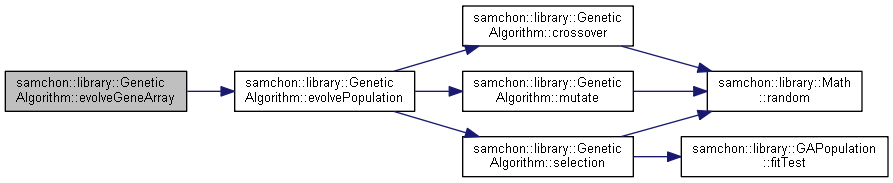Here is the caller graph for this function: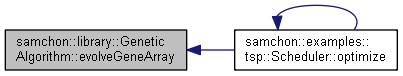template<typename GeneArray, typename Compare = std::less<GeneArray>>
 auto samchon::library::GeneticAlgorithm< GeneArray, Compare >::evolvePopulation ( std::shared_ptr< Population > population ) const -> std::shared_ptr
inline

Evolve population, a mass of GeneArray(es)

Parameters
 population An initial population

Definition at line 129 of file GeneticAlgorithm.hpp.

Here is the call graph for this function: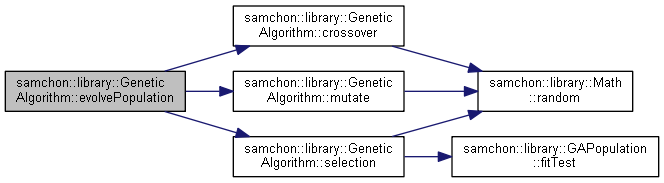Here is the caller graph for this function: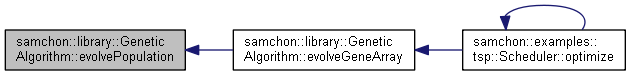template<typename GeneArray, typename Compare = std::less<GeneArray>>
 auto samchon::library::GeneticAlgorithm< GeneArray, Compare >::selection ( std::shared_ptr< Population > population ) const -> std::shared_ptr
inlineprivate

Select the best GeneArray in population from tournament.

Selection is the stage of a genetic algorithm in which individual genomes are chosen from a population for later breeding (using crossover operator). A generic selection procedure may be implemented as follows:

1. The fitness function is evaluated for each individual, providing fitness values, which are then normalized. Normalization means dividing the fitness value of each individual by the sum of all fitness values, so that the sum of all resulting fitness values equals 1.
2. The population is sorted by descending fitness values.
3. Accumulated normalized fitness values are computed (the accumulated fitness value of an individual is the sum of its own fitness value plus the fitness values of all the previous individuals). The accumulated fitness of the last individual should be 1 (otherwise something went wrong in the normalization step).
4. A random number R between 0 and 1 is chosen.
5. The selected individual is the first one whose accumulated normalized value is greater than R.
Parameters
 population The target of tournament
Returns
The best genes derived by the tournament

Definition at line 187 of file GeneticAlgorithm.hpp.

Here is the call graph for this function: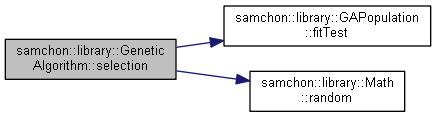Here is the caller graph for this function: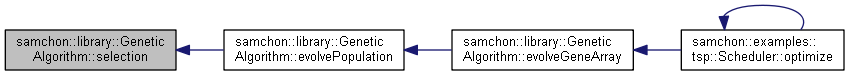template<typename GeneArray, typename Compare = std::less<GeneArray>>
 auto samchon::library::GeneticAlgorithm< GeneArray, Compare >::crossover ( std::shared_ptr< GeneArray > & parent1, std::shared_ptr< GeneArray > & parent2 ) const -> std::shared_ptr
inlineprivate

Create a new GeneArray by crossing over two GeneArray(s)

crossover is a genetic operator used to vary the programming of a chromosome or chromosomes from one generation to the next. It is analogous to reproduction and biological crossover, upon which genetic algorithms are based.

Cross over is a process of taking more than one parent solutions and producing a child solution from them. There are methods for selection of the chromosomes.

Parameters
 parent1 A parent sequence listing parent2 A parent sequence listing

Definition at line 219 of file GeneticAlgorithm.hpp.

References samchon::library::Math::random().

Here is the call graph for this function: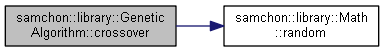Here is the caller graph for this function: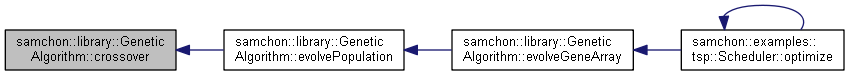template<typename GeneArray, typename Compare = std::less<GeneArray>>
 virtual void samchon::library::GeneticAlgorithm< GeneArray, Compare >::mutate ( std::shared_ptr< GeneArray > individual ) const
inlineprotectedvirtual

Cause a mutation on the GeneArray.

Mutation is a genetic operator used to maintain genetic diversity from one generation of a population of genetic algorithm chromosomes to the next. It is analogous to biological mutation.

Mutation alters one or more gene values in a chromosome from its initial state. In mutation, the solution may change entirely from the previous solution. Hence GA can come to better solution by using mutation.

Mutation occurs during evolution according to a user-definable mutation probability. This probability should be set low. If it is set too high, the search will turn into a primitive random search.

Note

Muttion is pursuing diversity. Mutation is useful for avoiding the following problem.

When initial set of genes(GeneArray) is far away from optimail, without mutation (only with selection and crossover), the genetic algorithm has a tend to wandering outside of the optimal.

Genes in the GeneArray will be swapped following percentage of the mutationRate.

Parameters
 individual A container of genes to mutate
mutationRate;

Definition at line 294 of file GeneticAlgorithm.hpp.

Here is the call graph for this function: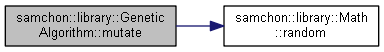Here is the caller graph for this function: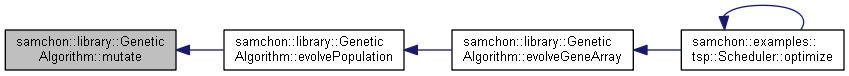## Member Data Documentation

template<typename GeneArray, typename Compare = std::less<GeneArray>>
 bool samchon::library::GeneticAlgorithm< GeneArray, Compare >::unique
protected

Whether each element (Gene) is unique in their GeneArray.

Definition at line 69 of file GeneticAlgorithm.hpp.

template<typename GeneArray, typename Compare = std::less<GeneArray>>
 double samchon::library::GeneticAlgorithm< GeneArray, Compare >::mutationRate
protected

Rate of mutate ocurrence.

The mutationRate determines the percentage of occurence of mutation in a GeneArray.

Note
• When mutationRate is too high, it is hard to ancitipate studying on genetic algorithm.
• When mutationRate is too low and initial set of genes(GeneArray) is far away from optimal, the evolution tends to wandering outside of he optimal.

Definition at line 82 of file GeneticAlgorithm.hpp.

template<typename GeneArray, typename Compare = std::less<GeneArray>>
 size_t samchon::library::GeneticAlgorithm< GeneArray, Compare >::tournament
protected

Size of tournament in selection.

Definition at line 87 of file GeneticAlgorithm.hpp.

The documentation for this class was generated from the following file: